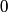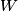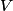## Solution to 1986 Problem 31

 Initially, when the voltage of the collecter is$0$, there is a current measured in the ammeter assuming that the photon energy is greater than the work function$W$. As the voltage$V$ is lowered, the electrons are decelerated by the electric field between the photocathode and the collector. So, when the electrons reach the collector, they have a smaller velocity and so the measured current is 0. Eventually, when the voltage$V$ is lowered enough, the electrons reaching the collector have a velocity of exactly$0$, so no current is measured. This occurs when the given equation is satisfied. Therefore, answer (A) is correct.